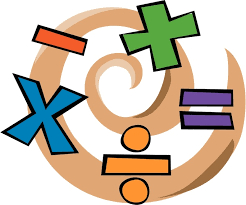# Sum or difference

Illustrate the sum or difference of the following expressions
1. 4/8+1/2=
2. 5/6-1/4=
3. 4-3/6=
4. 3/4+1/3=
5. 9/10-2/5=

a =  1
b =  7/12
c =  7/2 = 3 12
d =  13/12 = 1 112
e =  1/2

### Step-by-step explanation:Did you find an error or inaccuracy? Feel free to write us. Thank you!

Tips for related online calculators
Need help calculating sum, simplifying, or multiplying fractions? Try our fraction calculator.